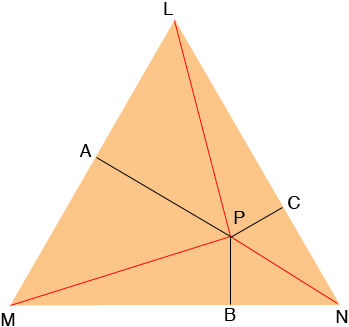SEARCH HOMEMath Central Quandaries & QueriesQuestion from Bibi, a student: An equilateral triangle has a point P inside it. PA, PB, PC are the perpendiculars from point P to the three sides of the triangle. Help me show that PA+PB+PC is the same no matter where the point P is..?Hi Bibi,I called the triangle LMN and joined P to each of the vertices. Hence triangle LMN is partitioned into three triangles, LMP, NP and NLP. What are the areas of these three triangles?

HarleyMath Central is supported by the University of Regina and The Pacific Institute for the Mathematical Sciences.